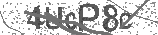﻿ Fractions on a Number Line Song for 3rd Grade & 4th Grade | Safe Videos for Kids
Welcome

# Fractions on a Number Line Song for 3rd Grade & 4th Grade

Thanks! Share it with your friends!

URL

You disliked this video. Thanks for the feedback!

Sorry, only registred users can create playlists.URLFind Related Videos  added
470 Views## Description

Enjoy our growing library of math videos at https://www.numberock.com ◀ Safe Link

1. VIDEO'S LESSON PLAN: COMING SOON!

2. NUMBEROCK DIGITAL LIBRARY 70% OFF: https://www.teacherspayteachers.com/Product/Math-Worksheets-More-NUMBEROCK-Musical-Math-Workbook-and-Activities-2200780 ◀ Safe Link

SUMMARY:

Join Benny in his school where he is investigating fractions on number lines. He gets some help from his friend Thania, and then shows his stuffed animal how to write 1/3 on the number line. While doing his homework later in the evening, Benny gets stuck on a problem about plotting a fraction on a number line that is greater than one, so he texts Thania to get some help.

LYRICS: US Coins

I asked my friend if she could help me find
where to write one-half on the number line.
She said, “First draw a line with two arrows.”
Then she sang a song; here’s how it goes:

Zero to one is the whole that we split apart.
The denominator tells us into how many parts.
Make a line that starts from zero to the first mark,
and one-half is what you’ll find when you get that far.

My stuffed animal can’t talk, but I thought I heard,
“Hey, Ben, on the number line, where’s one-third?”
I said, “First, draw a line with two arrows.
And hey! I know a cool tune; here’s how it goes.”

Zero to one is the whole that we split apart.
The denominator tells us into how many parts.
Make a line that starts from zero to the first mark,
and one-third is what you’ll find when you get that far.

Then I texted my friend, “What’s to be done
when the numerator isn’t one?”
She said, “Let’s try to find five over nine
on the number line in - like - no time.
Simply partition nine parts from zero to one.
Starting from zero, count five, and at five-ninths we arrive.”

Zero to one is the whole that we split apart.
The denominator tells us into how many parts.
Make a line that starts from zero to the fifth mark,
and five-ninths is what you’ll find when you get that far.

Let’s use the number line to describe this pie.
First, count the total pieces... there are five.
Five’s the denominator (all the parts), so five goes underneath the fraction bar.
This number line goes from zero to two,
but the fraction’s less than one, so here’s what to do:
Make five equal spaces from zero to one.
Now the final step before we’re done:
The numerator is the one piece that’s mine.
After the first space I’ll write one-fifth under the line.
Just remember this one tip in your minds:
Always count the spaces - never the lines.

Zero to one is the whole that we split apart.
The denominator tells us into how many parts.
Make a line that starts from zero to the first mark,
and one-fifth is what you’ll find when you get that far.

This video addresses the following Common Core Standards:
CCSS.Math.Content.3.NF.A.2
Understand a fraction as a number on the number line; represent fractions on a number line diagram.
CCSS.Math.Content.3.NF.A.2.a
Represent a fraction 1/b on a number line diagram by defining the interval from 0 to 1 as the whole and partitioning it into b equal parts. Recognize that each part has size 1/b and that the endpoint of the part based at 0 locates the number 1/b on the number line.
CCSS.Math.Content.3.NF.A.2.b
Represent a fraction a/b on a number line diagram by marking off a lengths 1/b from 0. Recognize that the resulting interval has size a/b and that its endpoint locates the number a/b on the number line.

http://www.NUMBEROCK.com is a provider of educational videos for kids which was founded by an innovative 5th Grade teacher who envisioned a new kind of classroom where students got energized for math class. Parents of his students even noticed their children singing mathematics songs around the dinner table well beyond school hours.

Seeing his students so enthusiastic when it was time for math was welcome, to say the least! But the epiphanous moment was when students were singing math songs all-day long like they were in the American Top 40!

We'll be releasing new songs and videos regularly until eventually addressing all the math skills and concepts that a Common Core Curriculum demands from 1st Grade to 2nd Grade, and even 5th Grade to 6th Grade.

Our main goal is to make teaching and learning math more fun and more interactive. SchoolHouse Rock! has done this in other subjects for us in the previous generations.Be the first to comment